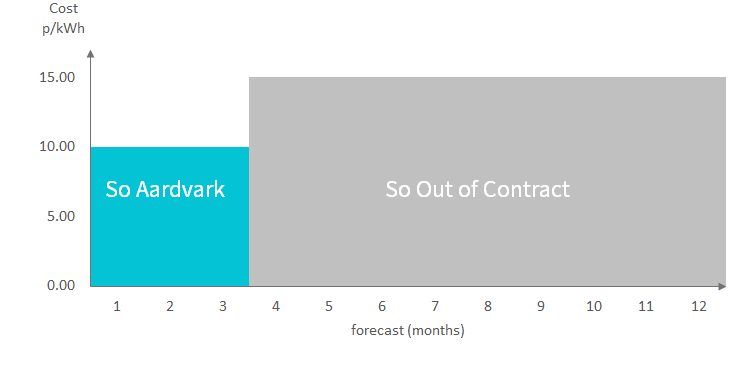## Estimated Annual Cost

### What is an Estimated Annual Cost

Your Estimated Annual Cost is a prediction of how much your energy use will cost over the next 12 months.

Your Estimated Annual Cost, sometimes called a Personal Projection, was introduced by Ofgem in 2014 as a tool to give customers a rough idea of what they should expect to spend on their energy and it must be shown on each statement we produce for you. The method for calculating this is provided by Ofgem.

Your Estimated Annual Cost is just an estimate for the future and does not include any credit / debit balance you may have on your account.

### How is my Estimated Annual Cost calculated

If you are on a tariff that is due to end in the next 12 months we are required to assume that you will move onto our So Out of Contract tariff (our standard variable tariff) when your current tariff expires. So if your current tariff expires in 3 months, your calculation will be based on 3 months on your existing tariff and 9 months (the remaining time) on our So Out of Contract tariff.Example

Assuming that your estimated electricity usage is 2,500 kWh and gas 14,000 kWh, So Aardvark tariff rates are (gas unit rate 3.00p/kWh, electricity unit rate 10.00p/kWh, Standing charge for both is 18.00p/day) and So Out of Contract tariff rates are (gas unit rate 3.50p/kWh, electricity unit rate 11.00p/kWh, Standing charge for both is 18.00p/day)

To calculate your Estimated Annual Cost:

Forecast electricity costs 3 months on So Aardvark tariff = (2,500 x 3/12) x 10.00p + (365 x 3/12) x 18.00p = £78.93
Forecast electricity costs 9 months on So Out of Contract tariff = (2,500 x 9/12)x 11.00p + (365 x 9/12) x 18.00p = £255.53
Forecast gas costs 3 months on So Aardvark tariff = (14,000 x 9/12) x 3.00p + (365 x 3/12) x 18.00p = £121.43
Forecast gas costs 9 months on So Out of Contract tariff = (14,000 x 9/12) x 3.50p + (365 x 9/12) x 18.00p = £416.78

Estimated Annual Cost (total) = £78.93 + £255.53 + £121.43 + £416.78 = £872.67

### Could you pay less

Ofgem requires us to tell you if we have another equivalent tariff (i.e. a 12 month fixed for another 12 month fixed) that’s cheaper than the one you’re on. You’ll see it on ‘Could you pay less’ section on the first page of your monthly statement.

The way we compare tariffs is by taking your Estimated Annual Cost (per fuel) and compare it to the 12 month costs on our latest tariffs. If the new tariff costs less than your Estimated Annual Cost, we'll tell you and by how much.

Using the example above, and our latest tariff rates (gas unit rate 2.50p/kWh, electricity unit rate 9.50p/kWh, Standing charge for both is 18.50p/day)

Estimated Annual Cost cost for electricity = £78.93 + £255.53 = £334.46
Forecast cost for electricity on the new tariff = 2,500 x 9.50p + 365 x 18.50p = £305.03
The amount we would show you saving by switching your electricity tariff is = £334.46 - £305.03 = £29.44

Estimated Annual Cost cost for gas = £121.43 + £416.78 = £538.21
Forecast cost for electricity on the new tariff = 14,000 x 2.50p + 365 x 18.50p = £417.53
The amount we would show you saving by switching your gas tariff is = £538.21 - £417.53 = £120.69

Cookies help us deliver our services. By using our services, you agree to our use of cookies.